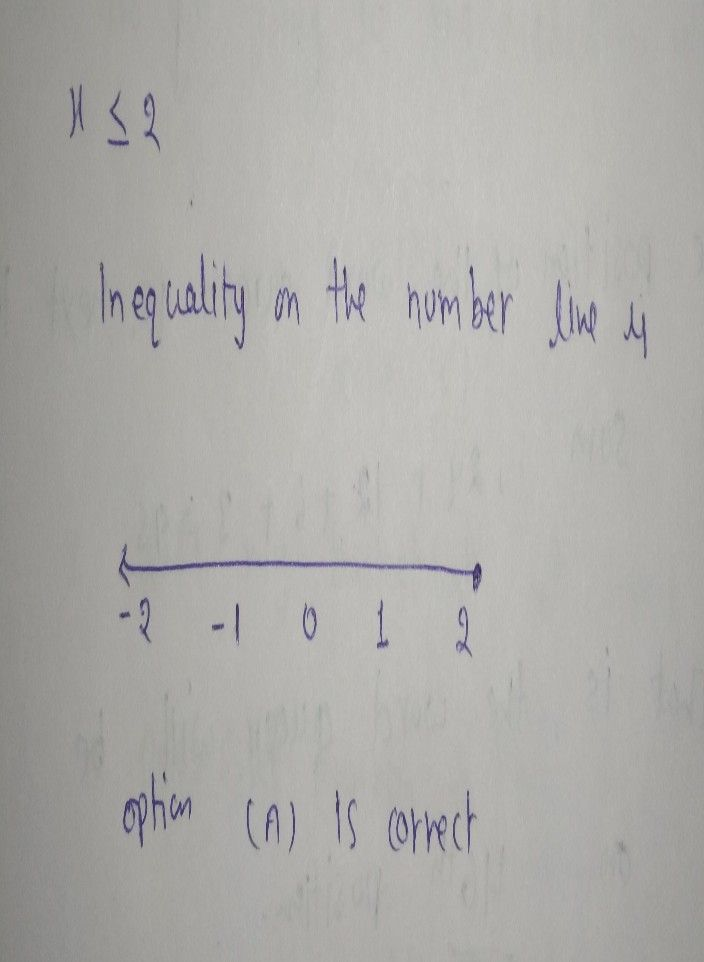Symbol
Problem$13$ Graph the following inequality on the number line. $x<2$ $○$ $-2$ $-1$ 21 $○$ $\bar{-2}$ $-1$ 2. $○$ $00$ $05$ $10$ $15$ $20$ $○$ $z0$ 30 40 50 60
7th-9th grade
Algebra
SolutionQanda teacher - AskSubhashhey dear feel free to ask queries
please evaluate the answer if you satisfied
Please give 3000 coins as thank you gift this will not reduce your coins
happy learning ☺️Student
thank you